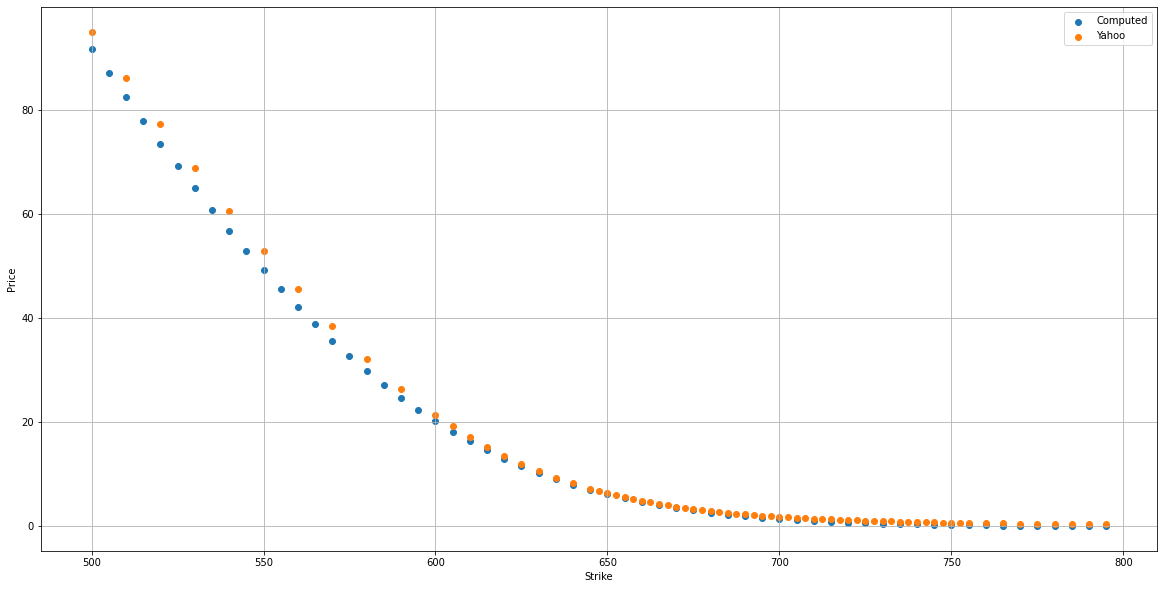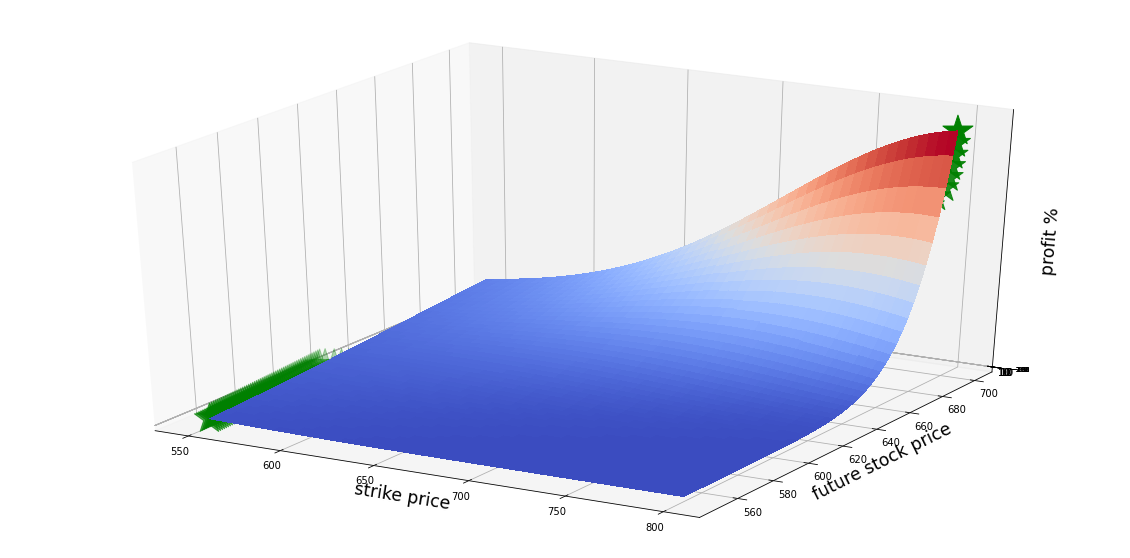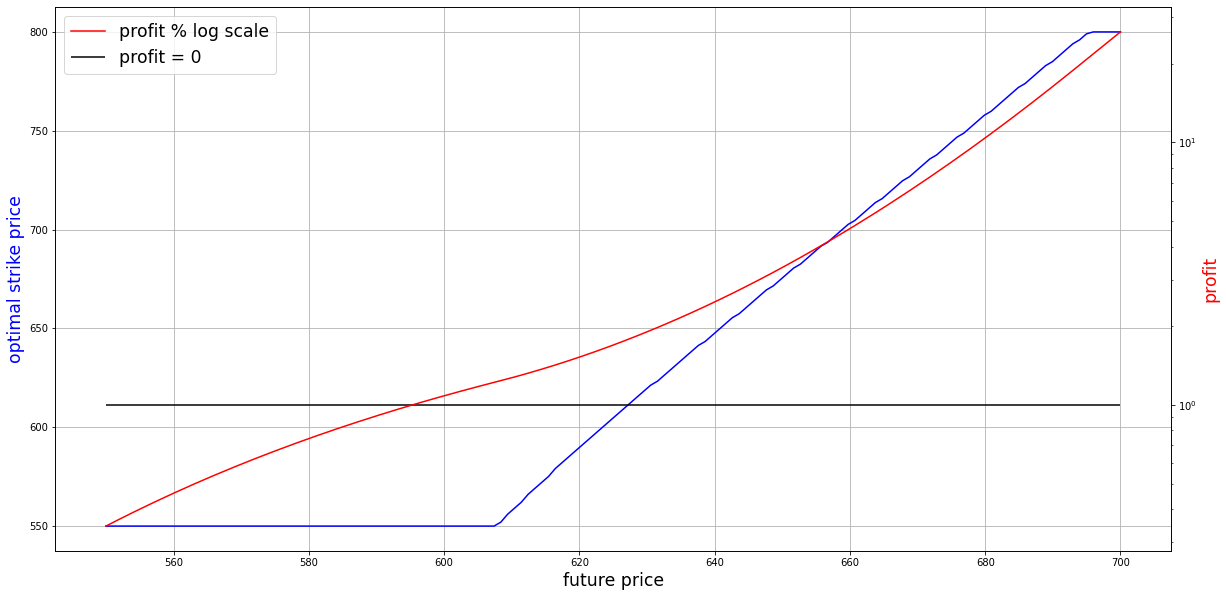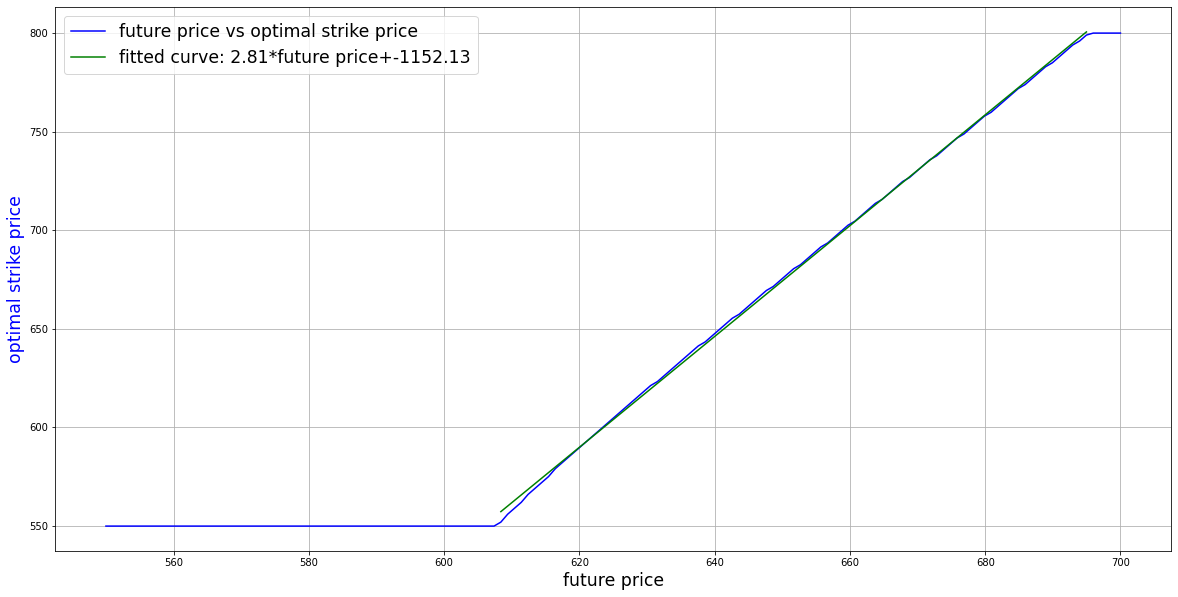# Optimal Option Strike Price vs Future Price

This post we studies the profit of call options for different strike price and price change. The goal is for the given current price and volacity level, expiration date and ticket, find the most profitable strike price for different future price change. Please read Option Pricing Model for background knowledge the option pricing.

# Background

For simplicity, we use the Black Scholes call option formula here, which is calculated by multiplying the stock price by the cumulative standard normal probability distribution function.

\begin{align*} C&=S_t \mathcal{N}(d_1)-Ke^{-rt}\mathcal{N}(d_2) \\ d_1&=\frac{\ln{\frac{S_t}{K}}+(r+\frac{\sigma_v^2}{2})t}{\sigma_s\sqrt{t}} \\ d_2&=d_1-\sigma_s\sqrt{t} \end{align*}

where

• C=Call option price
• S=Current stock (or other underlying) price
• K=Strike price
• r=Risk-free anual interest rate
• t=Years to maturity
• $\mathcal{N}$=cumulative density function of normal distribution

The profit of buying and then selling the option can then be written as:

$p(K,s_t)=\frac{C(K,s_t)}{C(K,s_0)}$

# Parameters

Those are parameters:

• current price is $$589\frac{74}{100}$$
• volatility is $$\frac{55}{100}$$, which is found from GraphVega
• anual interest rate is $$\frac{4}{100}$$

The figure belows shows the compute option price vs yahoo finance data. Check my previous post for how to fetch option data from yahoo finance via Pandas. The figure shows a good match between computed price vs real price.# Experiment

Let assume we buy an option which will expire in two weeks from now (May 28th 2021), the figure below plots the expected profit (%) vs strike price and predicted stock price in one week from now.The relationship of optimal strike price and profit vs future price is shown as below. When future price is below 610, your strike price should be as low as possible. When the future price is below 610, there is no way to make profit.In fact there is a linear relationship between optimal strike price and future price, which is strike price = 2.81 * future price - 1152Written on May 15, 2021